Adding + arithmetic progression - examples

1. Octahedron - sumOn each wall of a regular octahedron is written one of the numbers 1, 2, 3, 4, 5, 6, 7 and 8, wherein on different sides are different numbers. For each wall John make the sum of the numbers written of three adjacent walls. Thus got eight sums, which also.
2. AVG of INT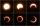What is the average of the integers from 9 throuht 52 inclusive?
3. Cinema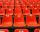Cinema auditorium is built for 3300 people. The first row is planned for 36 seats and each next gradually 4 more. How many rows of seats will have auditorium?
4. Workman - shift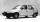The worker produces 300 components per shift. How many components would be produced in 18 shift, if his performance gradually increased every shift by 3 components?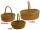Worker knits the baskets which then sells in the market. He maked seven baskets on Monday and one more each day than he did in the previous day. How many times more baskets did he make till the following Monday evening compared to the first day when he di
6. Chocholate pyramid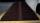How many chocolates are in the third shelf when at the 8th shelf are 41 chocolates in any other shelf is 7 chocolates more the previous shelf.
7. Series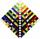Your task is express the sum of the following arithmetic series for n = 14: S(n) = 11 + 13 + 15 + 17 + ... + 2n+9 + 2n+11
8. Cinema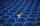How many rows of seats are in the stands, if you know that the first row has 24 seats and the last 50 seats and each subsequent row has two seats more than row before? How many seats are in the stands?
9. Three ints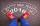The sum of three consecutive integers is 2016. What numbers are they?
10. Consecutive numbersSum of ten consecutive numbers is 105. Determine these numbers (write first and last).
11. Students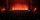In the front row sitting three students and in every other row 11 students more than the previous row. Determine how many students are in the room when the room is 9 lines, and determine how many students are in the seventh row.
12. Saving per cents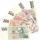The first day I save 1 cent and every next day cent more. How many I saved per year (365 days)?
13. Difference AP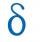Calculate the difference of arithmetic progression if the sum of its first 19 members Sn = 8075 and the first member is a1 = 20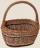Determine how many apples are in baskets when in the first basket are 4 apples, and in any other is 29 apples more than the previous, and we have eight baskets.What is the sum of the first two members of the aritmetic progression if d = -4.3 and a3 = 7.5?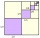Abdul takes a loan of 200000 from Ali and agrees to repay in number of instalment, each instalment begin with the 2nd exceeding the previous one by 1000, if the first instalment is 500, find how many instalment will be necessary to be wipe out the loan? CFind the sum of all natural numbers from 1 and 100, which are divisible by 2 or 5